# VBA Subroutine e Function

VBA has two types of procedures for creating and executing codes: Subroutine (or simply Sub) and Function.

So far, the examples in this tutorial have always been tied to a Sub. From here on, we will continue to assume that it is a Sub unless it is explicit that it is a Function.

## Subroutine

A Sub can be used to execute another Sub:

    Sub sub_main()
sub_auxiliary1
sub_auxiliary2
End Sub
Sub sub_auxiliary1()
MsgBox "One Sub executing another Sub"
End Sub
Sub sub_auxiliary2()
MsgBox "One Sub executing a second Sub"
End Sub


For this and further examples, just run the sub_main. By clicking anywhere between the line Sub sub_main() and the first End Sub.

A Sub can also accept an argument:

    Sub sub_main()
sub_argument(10) '10 is an argument
End Sub
Sub sub_argument(x as Integer)
MsgBox x
End Sub


You can assign an argument to the Sub using a single space:

    sub_argument 10 '10 is an argument


Or multiple arguments:

    Sub sub_main()
Dim score As Single
Dim student As String

score=10
student="Paulo"
Call sub_argument (score, student) 'score is an argument, student is another argument
End Sub
Sub sub_argument(s_exam as Single, name as String)
MsgBox name & "'s score was " & s_exam
End Sub


To have more than one argument into a Sub, you can use the Call instruction before the name of the Sub you want to call.

You can also execute a Sub with multiple arguments omitting the Call instruction and the parentheses:

sub_argument score, student

Have the practice of declaring the variables in the same data types that the Sub requires as arguments.
E.g.score and s_exam.

Subs can also be accessed from different modules: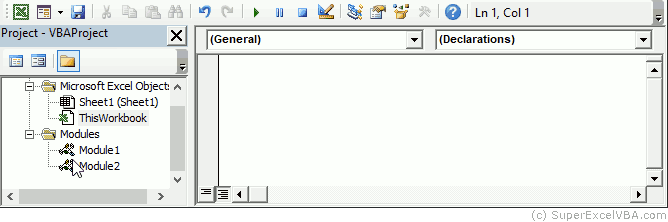## Types of Sub

The types of Sub available are Private and Public:

• Public Sub: Creates a public procedure that can be accessed by any procedure of any module, and is displayed in the Macros (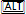+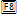).
• Private Sub: Creates a private procedure that can be accessed only by procedures of the same module, and is not displayed in the Macros (+).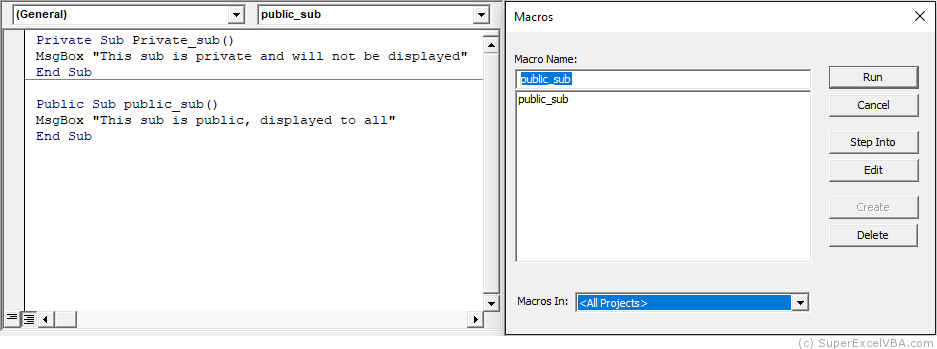An undefined Sub is considered Public by VBA.

## Functions

Functions work similarly to Subroutines with arguments. The main difference is that the Function will necessarily return a value and should be called by some other procedure.

    Sub sub_main()
result = multiply_2(10)
Msgbox result
End Sub
Function multiply_2(x As Single)
multiply_2 = x*2
End Function


It is not possible to pass arguments to a function through the single space if it is being associated with a variable.

    result = multiply_2 10 'Will result in error!


Note that:

MsgBox resultado 'It will not result in an error because there is no association.


You can not run a Function by itself. It is necessary to be called by another procedure.
E.g. Sub sub_main().

Once defined, functions can be used normally by the user in the Excel spreadsheet environment. Just call by the name of the function, as with any other predefined function (SUM, AVERAGE, etc.).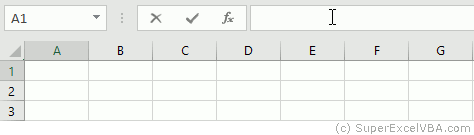We can also define in the declaration the type of result that the Function will return:

  Function multiply_2(x As Single) As Integer
multiply_2 = x*2
End Function


Note that now the function will only return rounded results, given the return specification As Integer.

There are many predefined functions in VBA. MsgBox is one of them.

        Msgbox "This should be shown" 'MsgBox works without the parentheses
Msgbox ("This should be shown") 'and works with the parentheses


## Naming a Function

When you create a function it needs to be followed by a name that must comply with the following rules:

• It must initiate with an alphabetical character
• It must not contain especial characters, such as: #, \$, %, &, !
• It must not contain whitespace characters, dots or commas
• It must not be a VBA reserved word like: And, Or, Sub

## Function Errors

Question: What if the user inserts unexpected values into my function?

Answer: Create an error to warn him.

This procedure can be done through the predefined CVErr function, which uses an integer value to specify an error.

The possible value it accepts are:

• 2000 (ou xlErrNull) which returns a #NULL! error
• 2007 (ou xlErrDiv0) which returns a #DIV/0 error
• 2015 (ou xlErrValue) which returns a #VALUE! error
• 2023 (ou xlErrRef) which returns a #REF! error
• 2029 (ou xlErrName) which returns a #NAME? error
• 2036 (ou xlErrNum) which returns a #NUM! error
• 2042 (ou xlErrNA) which returns a #N/A error

Compare how an User Defined Function (UDF) to calculate the area of a triangle should be changed to apply the CVErr

Function AreaTriangle(Base As Single, Height As Single) As Double
'As Double above specifies the return type of AreaTriangle
AreaTriangle = (Base * Height) / 2

End Function


Negative values are not expected for the area calculation, so let's create an error if the user enters them:

Function AreaTriangle(Base As Single, Height As Single) As Variant
'As Variant is defining the data type of AreaTriangle

If Base < 0 Or Height < 0 Then
AreaTriangle = CVErr(2036)
Else
AreaTriangle = (Base * Height) / 2
End If

End Function


In this way, if the user inserts negative values, the following error will appear in the cell of the function: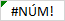The data type of CVErr(2036) is Error. This data type besides not being common is also incompatible with numeric data (that will be necessary for the calculation of the area). Therefore we assign to AreaTriangle the data type Variant. In this way, AreaTriangle will accept both the Error data type, if it occurs, and the Double data type, which will be returned if the calculation proceeded properly.

## Sub VS Function

• Subroutines do not return values if called. Functions return values
• Subroutines can be assigned to buttons and shapes in Excel, Functions can't
• Functions created in VBA can be used in your Excel worksheet

By default the predefined Excel functions are named in all capital letters.

Mixing uppercase and lowercase letters is a good way to differentiate from Excel and make explicit the functions that were created by the user.